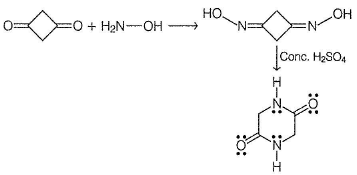Courses

# Test: Beckmann Rearrangement

## 17 Questions MCQ Test Chemistry Class 12 | Test: Beckmann Rearrangement

Description
This mock test of Test: Beckmann Rearrangement for Class 12 helps you for every Class 12 entrance exam. This contains 17 Multiple Choice Questions for Class 12 Test: Beckmann Rearrangement (mcq) to study with solutions a complete question bank. The solved questions answers in this Test: Beckmann Rearrangement quiz give you a good mix of easy questions and tough questions. Class 12 students definitely take this Test: Beckmann Rearrangement exercise for a better result in the exam. You can find other Test: Beckmann Rearrangement extra questions, long questions & short questions for Class 12 on EduRev as well by searching above.
QUESTION: 1

### Only One Option Correct Type Direction (Q. Nos. 1-7) This section contains 7 multiple choice questions. Each question has four choices (a), (b), (c) and (d), out of which ONLY ONE is correct. Q.  Which gives more than one amides on treatment with NH2OH followed by PCI5?

Solution:

Unsymmetrical carbonyls give more than one oximes with hydroxylamine, hence more than one amides in the subsequent Beckmann rearrangement.

QUESTION: 2

### Which oximes on treatment with concentrated H2SO4 undergo rearrangement to give single amide?

Solution:

Symmetrical oximes give single amide in Beckmann rearrangement.

QUESTION: 3

### What would be the major organic product in the following oxidation reaction?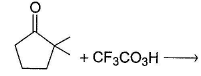Solution:

In BV oxidation, tertiary alkyl group has greater migrating aptitude than primary and secondary alkyl group.

QUESTION: 4

What is the final major product in the following reaction?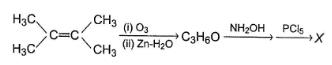Solution: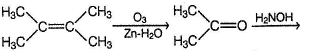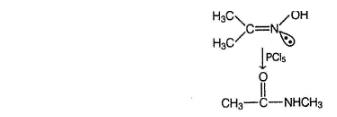QUESTION: 5

What is the major organic product in the following reaction?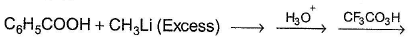Solution: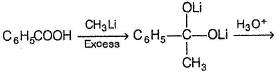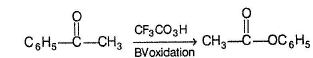QUESTION: 6

What is X in the following reaction?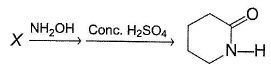Solution: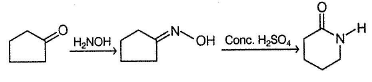QUESTION: 7

What is X in the following reaction?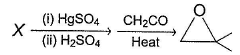Solution: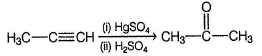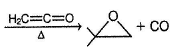*Multiple options can be correct
QUESTION: 8

One or More than One Options Correct Type

Direction (Q. Nos. 8-11) This section contains 4 multiple choice questions. Each question has four choices (a), (b), (c) and (d), out of which ONE or MORE THAN ONE are correct.

In the reaction given below,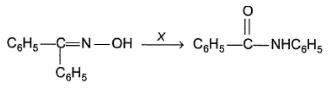Reagent X could be

Solution:

Beckmann rearrangement of oximes can be carried out with either PCI5 or Conc. H2SO4 or SOCI2.

*Multiple options can be correct
QUESTION: 9

Which reaction given below gives 2,2-dimethyl oxirane?

Solution:

(a) Addition of carbene at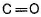gives oxirane.
(c) Epoxidation of alkene gives oxirane.

(d)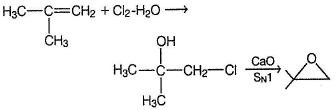*Multiple options can be correct
QUESTION: 10

In the reaction given below,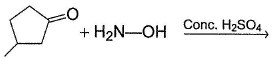Q.

expected product(s) is/are

Solution: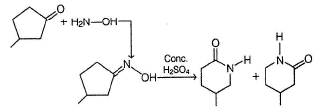*Multiple options can be correct
QUESTION: 11

The correct statainent(s) regarding the following reaction is/are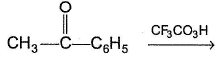Solution: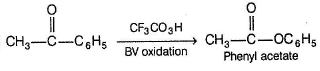QUESTION: 12

Comprehension Type

Direction (Q. Nos. 12-14) This section contains a paragraph, describing theory, experiments, data, etc.
Three questions related to the paragraph have been given. Each question has only one correct answer among the four given options (a), (b), (c) and (d).

Passage

Consider the following sequence of an organic synthesis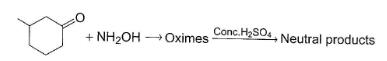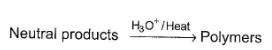Q.

If the starting compound has all its stereoisomers present, how many different oximes would be produced?

Solution: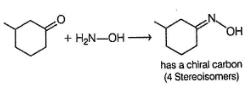QUESTION: 13

Consider the following sequence of an organic synthesis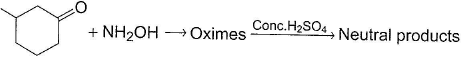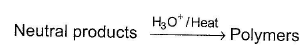Q.

Which is not a part of neutral products?

Solution: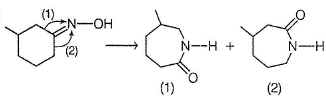(1) and (2) are the only rearrangement products.

QUESTION: 14

Consider the following sequence of an organic synthesisQ.

What is the possible repeating unit of polymer formed at the end of the reaction ?

Solution: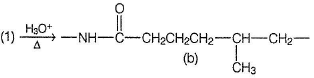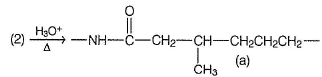*Answer can only contain numeric values
QUESTION: 15

One Integer Value Correct Type

Direction (Q. Nos. 15-17) This section contains 3 questions. When worked out will result in an integer from 0 to 9 {both inclusive).

In the reaction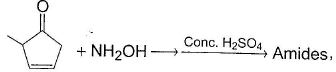Q.

how many different amides are expected?

Solution:

Both of pair below has one chiral carbon each, exist as a pair of enantiomers each.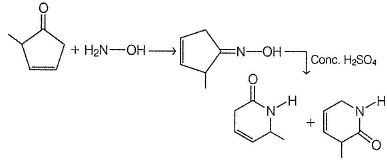*Answer can only contain numeric values
QUESTION: 16

In the reaction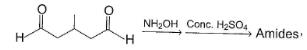Q.

how many different amides are expected?

Solution: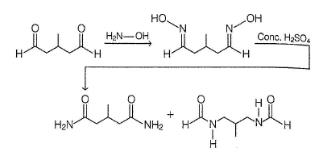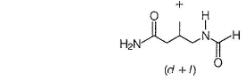*Answer can only contain numeric values
QUESTION: 17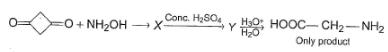Q.

How many lone pair of electrons are present in Y?

Solution: